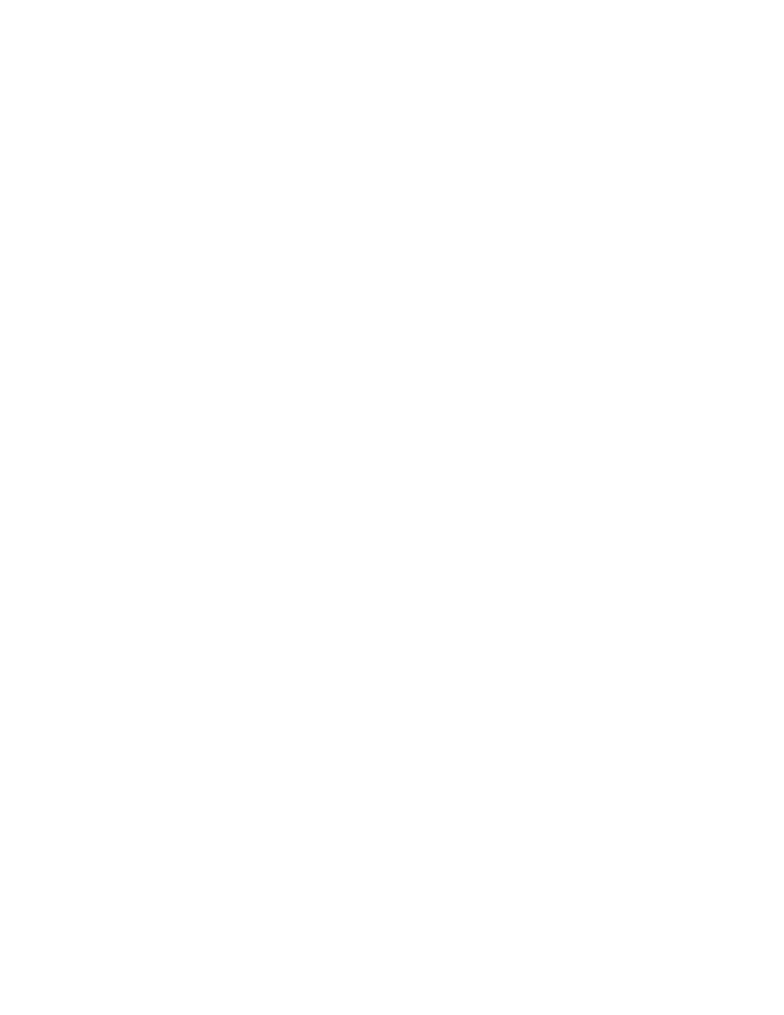Study Guides (390,000)
CA (150,000)
Western (10,000)
ECON (500)

# Economics 1021A/B Study Guide - Insulin, Economic Equilibrium, Inferior Good

Department
Economics
Course Code
ECON 1021A/B
Professor
Jeannie Gillmore

This preview shows page 1. to view the full 4 pages of the document.Econ 1021 Chap 4
Price Elasticity of Demand
Supply increase equilibrium price falls equilibrium quantity
increase
Large price change small quantity change
Small price change large quantity change
Price elasticity of demand units-free measure of responsiveness of
quantity demanded of a good to a change in price when all other inluences on
Calculating Elasticity of Demand
Price elasticity of demand = percentage change in quantity demanded
________________________________________________
percentage change in price
Express change in price as percentage of average price
Change in quantity demanded as percentage of avg quantity
Orig price \$20.50 new price \$19.50
Price change \$1 --> average price is \$20/pizza
Average Price and Quantity
Avg price/quantity most precise measurement of elasticity --> midpoint
b/w original and new
Price fall from 20.50 to 19.50 --> \$1 change is 4.9 percent of 20.50
2 pizza change in quantity is 22.2% of 9 pizzas
Price elasticity of demand is 22.2/4.9 = 4.5
Percentage and Proportions
Elasticity --> ratio of 2 percentage changes
When divide one percent by another 100s cancel
Percentage change is a proportionate change multiplied by 100
Proportionate change in price is delta p/p avg
Proportionate change in quan demanded is delta q/q avg
Units-Free Measure
Elasticity --> units free measure --> percentage change in each variable is
dependent of units in wihich variable is measured
Ratio of 2 percentages --> # w/o units
Minus Sign and Elasticity
Price of good rise --> quantity demanded decrease
b/c pos change in price brings neg change in quan demanded
price elasticity of demand = neg number